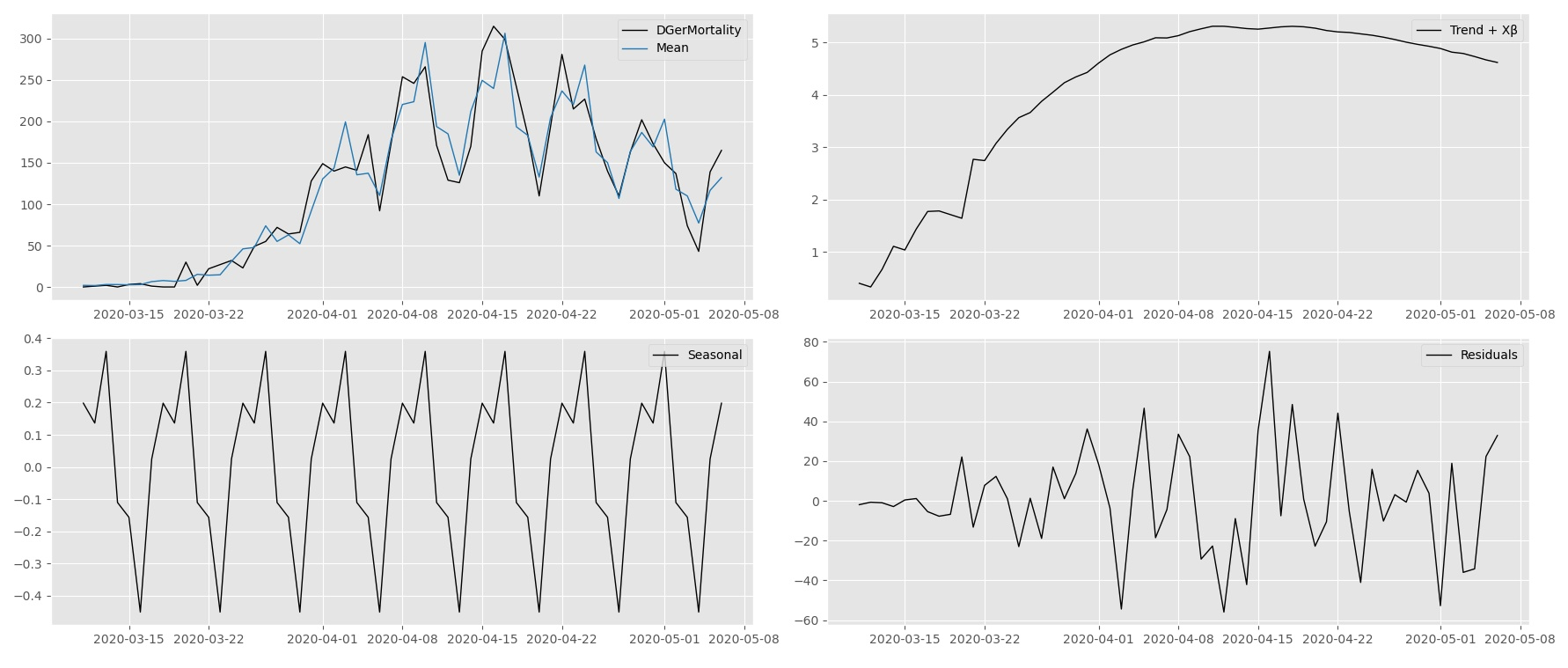### Score-driven methodology

We encourage you to read this section about the Kalman filter and State Space models first.

Dynamic Score models are a class of linear and non-linear estimators that can be used to analyse and forecast a wide range of time series. Score-driven models are so versatile that well-known models like ARMA and GARCH models, are subclasses of score-driven models. Furthermore, the score-driven model encompasses other well-known models autoregressive conditional duration (ACD) model, autoregressive conditional intensity (ACI) model, and Poisson count models with time-varying mean.

Score-driven models or Generalized Autoregressive Score models were proposed in their full generality in Creal, Koopman, and Lucas (2013) as developed at the time at VU University Amsterdam. Simultaneously, Harvey authored the book Dynamic Models for Volatility and Heavy Tails: With Applications to Financial and Economic Time Series (2013) in which the mechanism to update the parameters over time is the scaled score of the likelihood function.

### Mathematical formulation

In the class of score-driven models, the latent time-varying parameter vector $\alpha_t$ is updated over time using an autoregressive updating function based on the score of the conditional observation probability density function, see Creal, Koopman, and Lucas (2013) and Harvey (2013). The updating function for $\alpha_t$ is given by $\alpha_{t+1} = \omega + \sum_{i=1} ^p A_i s_{t-i+1} + \sum_{j=1} ^q B_j \alpha_{t-j+1},$ where $\omega$ is a vector of constants, $A$ and $B$ are fixed coefficient matrices and $s_t$ is the scaled score function which is the driving force behind the updating equation. The unknown coefficients $\omega$, $A$ and $B$ depend on the static parameter vector $\psi$. The definition of $s_t$ is $\qquad s_t = S_t \cdot \nabla_t, \qquad \nabla_t = \frac{\partial \, \log \, p(y_t | \alpha_t, \mathcal{F}_{t-1} ; \psi)}{\partial \alpha_t},$ for $t = 1,\ldots,n,$ and where $\nabla_{t}$ is the score vector of the (predictive) density $p(y_t | \alpha_t, \mathcal{F}_{t-1} ; \psi)$ of the observed time series $y = \left(y_1',\ldots,y_n' \right)'$. The information set $\mathcal{F}_{t-1}$ usually consists of lagged variables of $\alpha_t$ and $y_t$ but can contain exogenous variables as well. To introduce further flexibility in the model, the score vector $\nabla_{t}$ can be scaled by a matrix $S_t$. Common choices for $S_t$ are unit scaling, the inverse of the Fisher information matrix, or the square root of the Fisher inverse information matrix. The latter has the advantage of giving $s_t$ a unit variance since the Fisher information matrix is the variance matrix of the score vector. In this framework and given past information, the time-varying parameter vector $\alpha_t$ is perfectly predictable one-step-ahead. The score-driven model has three main advantages:

• the 'filtered' estimates of the time-varying parameter are optimal in a Kullback-Leibler sense.
• since the score-driven models are observation driven, their likelihood is known in closed-form.
• the forecasting performance of these models is comparable to their parameter-driven counterparts.

The second point emphasises that static parameters can be estimated in a straightforward way using maximum likelihood methods.The DGerMortality time series in the top left corner is decomposed into the time-varying components; Trend, Xβ, Seasonal, and Residuals.

The time series methodology of Time Series Lab - Dynamic Score Edition is well-founded in the academic literature and has appeared in highly respected academic journals. For an overview of score-driven publications, we refer to the www.gasmodel.com website. The score-driven methodology was developed independently at VU University Amsterdam and Cambridge University. Currently, the knowledge and experience of both universities have been combined and Professor S.J. Koopman (VU Amsterdam) and Professor A.C. Harvey (Cambridge) are part of the Time Series Lab team.

The Time Series Lab team has many years of experience in the field of time series analysis, both in academia and the industry. Time series research of the Time Series Lab team has been published in top statistical and econometrical journals for decades and it continues to this day. All this combined knowledge accumulates in the Time Series Lab software products.
The close connection between academia and the industry is established by positions held at major universities and research institutes with the combination of consultancy work for hedge funds, (central) banks, and marketing firms.

### Probability distributions

The most well-known probability distribution is the Normal, or Gaussian, distribution. Despite its popularity, many time series require a different distribution than the Normal distribution. Time Series Lab offers a variety of probability distributions and we are working to extent the library of distributions further.

Time Series Lab currently supports the following continuous probability distributions:
• Normal
• Student t
• Generalised Error Distribution
• Exponential Generalised Beta 2
• Exponential
• Weibull
And the following discrete probability distributions:
• Poisson
• Negative Binomial
• Bernoulli
• Skellam

# Bibliography

### References

Creal, D. D., S. J. Koopman, and A. Lucas (2013). Generalized autoregressive score models with applications. Journal of Applied Econometrics 28, 777–795.

Harvey, A. C. (2013). Dynamic Models for Volatility and Heavy Tails: With Applications to Financial and Economic Time Series. Econometric Series Monographs. Cambridge: Cambridge University Press.